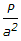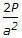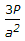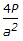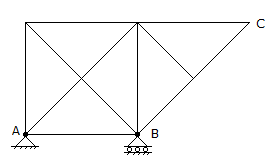# Civil Engineering - Theory of Structures

Exercise : Theory of Structures - Section 2
26.
A cantilever of length 2 cm and depth 10 cm tapers in plan from a width 24 cm to zero at its free end. If the modulus of elasticity of the material is 0.2 x 106 N/mm2, the deflection of the free end, is
2 mm
3 mm
4 mm
5 mm
6 mm
Explanation:
No answer description is available. Let's discuss.

27.
Pick up the correct statement from the following:
The bending stress in a section is zero at its neutral axis and maximum at the outer fibres
The shear stress is zero at the outer fibres and maximum at the neutral axis
The bending stress at the outer fibres, is known as principal stress
The planes of principal stresses are inclined at 45° to the neutral plane
All the above.
Explanation:
No answer description is available. Let's discuss.

28.
A simply supported rolled steel joist 8 m long carries a uniformly distributed load over it span so that the maximum bending stress is 75 N/mm2. If the slope at the ends is 0.005 radian and the value of E = 0.2 x 106 N/mm2, the depth of the joist, is
200 mm
250 mm
300 mm
350 mm
400 mm
Explanation:
No answer description is available. Let's discuss.

29.
A square column carries a load P at the centroid of one of the quarters of the square. If a is the side of the main square, the combined bending stress will be